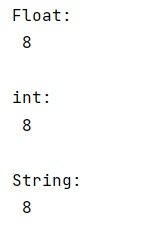# Size of data type using NumPy

Learn how to get the size of a data type using NumPy?
Submitted by Pranit Sharma, on February 09, 2023

NumPy is an abbreviated form of Numerical Python. It is used for different types of scientific operations in python. Numpy is a vast library in python which is used for almost every kind of scientific or mathematical operation. It is itself an array which is a collection of various methods and functions for processing the arrays.

## Getting the size of a data type using NumPy

In a programming language, data types are the particular format in which a value is being stored. A data type is a kind of data item, which represents what values it can take, the programming language used, or the operations that can be performed on it.

We can get the size of the data type in numpy using numpy.dtype.itemsize() but we need an instance of the dtype to get the item size.

Let us understand with the help of an example,

## Python code to get the size of a data type using NumPy

```# Import numpy
import numpy as np

# Display size of different data types
print("Float:\n",np.dtype(float).itemsize,"\n")

print("int:\n",np.dtype(np.int64).itemsize,"\n")

print("String:\n",np.dtype('O').itemsize,"\n")
```

Output: